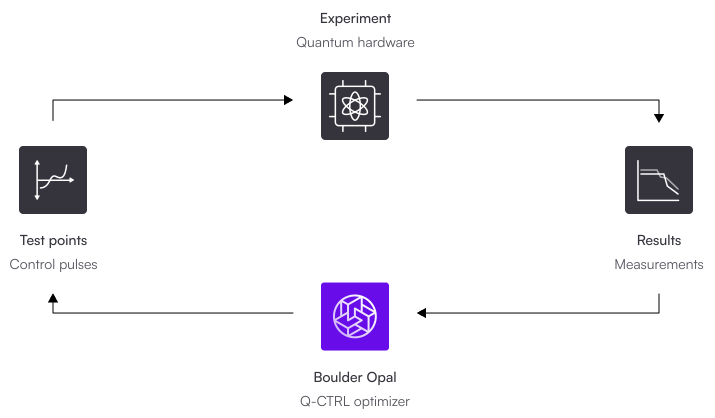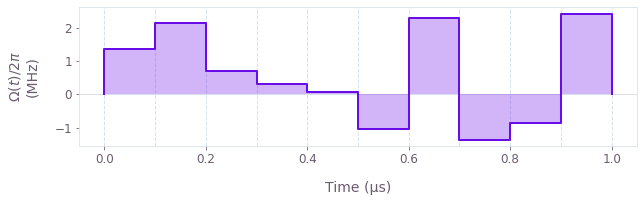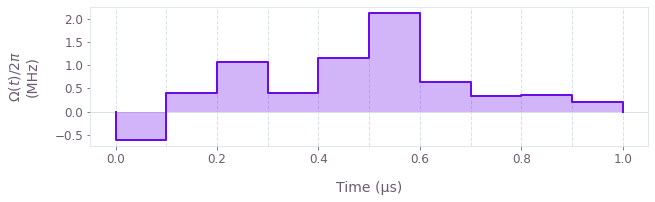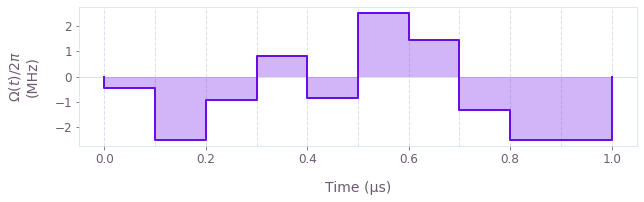•Boulder Opal
• User guides
• Automate closed-loop hardware optimization

# How to automate closed-loop hardware optimization

Closed-loop optimization without complete system models

Boulder Opal contains automated closed-loop optimization tools that do not require a complete understanding of the workings of your quantum system. These tools allow you to run optimizations incorporating input data rather than building a detailed model of your system.

This notebook will show how you can find optimized solutions using Boulder Opal's closed-loop optimization toolkit directly interacting with an experiment (here, simulated). It shows how to set up a closed-loop optimization with three different methods: Gaussian processes, simulated annealing, and covariance matrix adaptation evolution strategy (CMA-ES).

The Boulder Opal Toolkits are currently in beta phase of development. Breaking changes may be introduced.

## Closed-loop optimization framework

You can use the automated closed-loop optimizers to create a closed optimization loop where the optimizer communicates with the experimental apparatus without your direct involvement. In this kind of setting, your experimental apparatus produces an initial set of results, which it sends to the optimizer. Using this information, the optimizer produces a set of improved test points that it recommends back to the experimental apparatus. The results corresponding to these test points are resent to the optimizer, and the cycle repeats itself until any of the results has a sufficiently low cost function value, or until it meets any other ending condition that you imposed. This setup is illustrated in the figure below.## Summary workflow

### 1. Set up an interface with the experiment

A closed-loop optimization attempts to find a set of parameters that are capable of minimizing the value of a cost function. The parameters are real numbers that represent quantities that you can control and change from experiment to experiment. This means that the exact nature of the parameters depends on the problem that you are trying to solve, but in the context of quantum control they typically represent the values of a piecewise-constant control pulse.

From the result of each experiment, you must obtain a cost, a real number that represents a quantity that you want to minimize. The exact nature of the cost also depends on the problem you want to solve, but in the context of quantum control the cost will typically be an infidelity with respect to the ideal result of the operation.

During the course of the closed-loop optimization, the optimizer will generate test parameter sets in order to execute experiments with them. In order for the closed-loop optimization to interface with your experimental apparatus, you need to define a cost function which takes a set of parameters (as a NumPy array of shape (test_point_count, parameter_count)) and returns the associated cost of each set (as a 1D NumPy array of length test_point_count). The nature of this interface will depend on your experimental apparatus.

#### Establish experimental batching

In some cases it is advantageous to configure the optimizer to accept multiple test point/measurement pairs in each step—such as measurements with substantial communications latency on a cloud quantum computer. If your apparatus does not support this type of batching, you can achieve a simpler integration by using M-LOOP, which handles the optimization loop for you. You can learn more about how to integrate M-LOOP with Boulder Opal in this user guide.

### 2. Configure closed-loop optimization

#### Determine initial seed

Before starting the closed-loop optimization, you will need to collect a few initial results for the optimizer to use as a starting point. Create a collection of parameter sets that are valid for the problem that you are considering, and store them in a NumPy array. In the case of quantum control, this will typically mean a range of pulse shapes to subject the qubits to.

#### Select and initialize the optimizer

Create an object with the optimizer that you want to use, passing any necessary configuration for it. Details of all available Boulder Opal automated optimizers are available in our closed-loop optimization toolkit documentation.

### 3. Execute optimization

Provide to the qctrl.closed_loop.optimize function with the cost_function that you defined, the initial_test_parameters, the optimizer that you want to use, and the bounds for each parameter. The function will iterate calling the cost function and generating new test points to explore the parameter landscape until any of the convergence criteria are met.

import numpy as np
from qctrlvisualizer import plot_controls

from qctrl import Qctrl

# Start a Boulder Opal session
qctrl = Qctrl(verbosity="QUIET")

## Example: Designing an optimal control for a qubit subject to unknown control operators using Gaussian processes

Consider a qubit whose precise Hamiltonian is unknown to you. Specifically, suppose that you want to create an optimized X gate but your Hamiltonian contains unknown terms:

$$H(t) = \frac{\Omega(t)}{2} \left( \sigma_x + Q_\text{unknown} \right).$$

In the previous equation, $\Omega(t)$ define the control pulses, and $Q_\textrm{unknown}$ are extra unknown terms that appear when applying your control. This example shows how you can find the optimal pulse for this system without having to ever learn the form of this extra term.

### Set up an interface with the experiment

In a practical situation, you'll be obtaining the data from your experimental equipment. In this example, the experimental results are simulated using Boulder Opal. In either case, you'll need a function that accepts sets of parameters (the piecewise-constant values of $\Omega(t)$) and returns their corresponding infidelities with respect to the target X gate, which act as the cost. The function in the following code block implements this with input and output arrays with the appropriate shapes.

# Define standard deviation of the errors in the experimental results.
sigma = 0.01

# Define standard matrices.
sigma_x = np.array([[0, 1], [1, 0]], dtype=complex)
sigma_y = np.array([[0, -1j], [1j, 0]], dtype=complex)
sigma_z = np.array([[1, 0], [0, -1]], dtype=complex)

# Define control parameters.
duration = 1e-6  # s

# Create a random unknown operator.
rng = np.random.default_rng(seed=10)
phi = rng.uniform(-np.pi, np.pi)
u = rng.uniform(-1, 1)
Q_unknown = (
u * sigma_z + np.sqrt(1 - u**2) * (np.cos(phi) * sigma_x + np.sin(phi) * sigma_y)
) / 4

# Define simulation of a quantum experiment to use in the optimization loop.
def run_experiments(omegas):
"""
Simulates a series of experiments where controls omegas attempt to apply
an X gate to a system. The result of each experiment is the infidelity plus
a Gaussian error.

In your actual implementation, this function would run the experiment with
the parameters passed. Note that the simulation handles multiple test points,
while your experimental implementation might need to queue the test point
requests to obtain one at a time from the apparatus.
"""

# Create the graph with the dynamics of the system.
graph = qctrl.create_graph()
signal = graph.pwc_signal(values=omegas, duration=duration)
graph.infidelity_pwc(
hamiltonian=0.5 * signal * (sigma_x + Q_unknown),
target=graph.target(operator=sigma_x),
name="infidelities",
)

# Run the simulation.
result = qctrl.functions.calculate_graph(
graph=graph, output_node_names=["infidelities"]
)

# Add error to the measurement.
error_values = rng.normal(loc=0, scale=sigma, size=len(omegas))
infidelities = result.output["infidelities"]["value"] + error_values

# Return only infidelities between 0 and 1.
return np.clip(infidelities, 0, 1)

### Configure closed-loop optimization

#### Determine initial seed

After defining the experimental interface, you need to obtain a set of initial results. You will use these as the initial input for the automated closed-loop optimization algorithm. The distribution of the initial test points will influence the convergence of the algorithm, with the optimal distribution depending on the particular problem to be solved. Common choices are to sample a uniform distribution or a distribution centered about some point of interest (such as default or model-obtained values).

In this case we use 20 initial parameter sets, each one representing a pulse with a constant value. The values are uniformly spaced, with the first and last sets creating the desired gate if the unknown term was not present in the Hamiltonian.

# Define the number of test points obtained per run.
test_point_count = 20

# Define number of segments in the control.
segment_count = 10

# Define initial parameter set as constant controls with piecewise constant segments.
initial_test_parameters = (
(np.pi / duration)
* (np.linspace(-1, 1, test_point_count)[:, None])
* np.ones((test_point_count, segment_count))
)

#### Select and initialize the optimizer

This example uses the object qctrl.closed_loop.GaussianProcess to set up an automated closed-loop optimization that uses the Gaussian process method (GP). You can use analogous objects to perform the optimization with other methods, although the set of arguments will vary with the method.

This method tries to fit a Gaussian process model to the data provided. The optimizer object takes the length_scale_bounds: the bounds of the length scales of the model's Gaussian kernel, which are tuned while training the model during the optimization. Roughly speaking, the amount a parameter needs to change to impact the optimization cost should lie within these length scale bounds.

# Define length-scale bounds as a NumPy array.
length_scale_bounds = np.repeat([[1e-5, 1e5]], segment_count, axis=0)

# Create Gaussian Process optimizer object.
gp_optimizer = qctrl.closed_loop.GaussianProcess(
length_scale_bounds=length_scale_bounds, seed=0
)

### Execute optimization

The qctrl.closed_loop.optimize function will iterate executing the cost function with new test points until it converges to the target_cost or reaches the max_iteration_count.

# Define bounds for all optimization parameters.
bounds = np.repeat([[-1, 1]], segment_count, axis=0) * 5 * np.pi / duration

# Create random number generator for the simulation function.
simulation_seed = 5
rng = np.random.default_rng(simulation_seed)

# Run the closed-loop optimization.
gp_results = qctrl.closed_loop.optimize(
cost_function=run_experiments,
initial_test_parameters=initial_test_parameters,
optimizer=gp_optimizer,
bounds=bounds,
target_cost=3 * sigma,
cost_uncertainty=sigma,
)
Running closed loop optimization
----------------------------------------
Optimizer            : Gaussian process
Number of test points: 20
Number of parameters : 10
----------------------------------------

Calling cost function…
Initial best cost: 0.103

Running optimizer…
Calling cost function…
Best cost after 1 iterations: 0.036

Running optimizer…
Calling cost function…
Best cost after 2 iterations: 0.036

Running optimizer…
Calling cost function…
Best cost after 3 iterations: 0.036

Running optimizer…
Calling cost function…
Best cost after 4 iterations: 0.036

Running optimizer…
Calling cost function…
Best cost after 5 iterations: 0.036

Running optimizer…
Calling cost function…
Best cost after 6 iterations: 0.031

Running optimizer…
Calling cost function…
Best cost after 7 iterations: 0.031

Running optimizer…
Calling cost function…
Best cost after 8 iterations: 0.031

Running optimizer…
Calling cost function…
Best cost after 9 iterations: 0.023

Target cost reached. Stopping the optimization.

# Print optimized cost and plot optimized controls.
print(f"\nOptimized infidelity: {gp_results['best_cost']:.5f}")
plot_controls(
{
r"$\Omega(t)$": qctrl.utils.pwc_arrays_to_pairs(
duration, gp_results["best_parameters"]
)
}
)
Optimized infidelity: 0.02338## Example: Designing an optimal control for a qubit subject to unknown control operators using simulated annealing

In this section we employ the same model as above but use the object qctrl.closed_loop.SimulatedAnnealing to set up an automated closed-loop optimization that uses the simulated annealing (SA) process.

### Set up an interface with the experiment

As this example deals with the same problem as before, we will reuse run_experiments as the cost function, and will seed the SA optimizer with the same set of initial test points.

### Configure closed-loop optimization

The simulated annealing hyperparameters temperatures and temperature_cost control the overall exploration and greediness of the optimizer, respectively. More difficult optimization problems typically require higher temperatures because high fidelity controls tend to vary greatly from the initial guesses provided to the optimizer.

In real life problems, determining the optimal choice of temperatures and temperature_cost is generally not feasible or necessary. Some level of searching usually needs to be done on the part of the user. Here, the temperatures have been set to 400000 after testing temperatures of varying magnitude, that is, 400, 4000, 40000, .... Such a search is often easily parallelizable, and heuristically, temperatures one order of magnitude smaller than the provided bound tend to be a decent starting point for a search. Similar heuristics apply to the temperature_cost, where starting a grid search approximately one order of magnitude smaller than the range of the cost tends to be a decent starting point. For additional hyperparameter tuning methods, visit the Wikipedia article on Hyperparameter optimization.

sa_optimizer = qctrl.closed_loop.SimulatedAnnealing(
temperatures=np.repeat(4.0e5, segment_count), temperature_cost=0.25, seed=0
)

### Execute optimization

# Reset random number generator for simulation function.
rng = np.random.default_rng(simulation_seed)

sa_results = qctrl.closed_loop.optimize(
cost_function=run_experiments,
initial_test_parameters=initial_test_parameters,
optimizer=sa_optimizer,
bounds=bounds,
target_cost=3 * sigma,
cost_uncertainty=sigma,
)
Running closed loop optimization
----------------------------------------
Optimizer            : simulated annealing
Number of test points: 20
Number of parameters : 10
----------------------------------------

Calling cost function…
Initial best cost: 0.103

Running optimizer…
Calling cost function…
Best cost after 1 iterations: 0.087

Running optimizer…
Calling cost function…
Best cost after 2 iterations: 0.087

Running optimizer…
Calling cost function…
Best cost after 3 iterations: 0.024

Target cost reached. Stopping the optimization.

# Print optimized cost and plot optimized controls.
print(f"\nOptimized infidelity: {sa_results['best_cost']:.5f}")
plot_controls(
{
r"$\Omega(t)$": qctrl.utils.pwc_arrays_to_pairs(
duration, sa_results["best_parameters"]
)
}
)
Optimized infidelity: 0.02361## Example: Designing an optimal control for a qubit subject to unknown control operators using CMA-ES

In this section we employ the same model as in the previous examples, but using qctrl.closed_loop.Cmaes to set up a covariance matrix adaptation evolution strategy (CMA-ES) closed-loop optimization.

### Set up an interface with the experiment

As this example deals with the same problem as before, we will reuse run_experiments as the cost function, and will seed the CMA-ES optimizer with the same set of initial test points.

### Configure closed-loop optimization

As in the other examples, we create an object defining the optimization configuration, in this case, a qctrl.closed_loop.Cmaes.

# Define initialization object for the CMA-ES optimizer.
cmaes_optimizer = qctrl.closed_loop.Cmaes(seed=0)

### Execute optimization

# Reset random number generator for simulation function.
rng = np.random.default_rng(simulation_seed)

cmaes_results = qctrl.closed_loop.optimize(
cost_function=run_experiments,
initial_test_parameters=initial_test_parameters,
optimizer=cmaes_optimizer,
bounds=bounds,
target_cost=3 * sigma,
cost_uncertainty=sigma,
)
Running closed loop optimization
----------------------------------------
Optimizer            : CMA-ES
Number of test points: 20
Number of parameters : 10
----------------------------------------

Calling cost function…
Initial best cost: 0.103

Running optimizer…
Calling cost function…
Best cost after 1 iterations: 0.060

Running optimizer…
Calling cost function…
Best cost after 2 iterations: 0.035

Running optimizer…
Calling cost function…
Best cost after 3 iterations: 0.019

Target cost reached. Stopping the optimization.

# Print optimized cost and plot optimized controls.
print(f"\nOptimized infidelity: {cmaes_results['best_cost']:.5f}")
plot_controls(
{
r"$\Omega(t)$": qctrl.utils.pwc_arrays_to_pairs(
duration, cmaes_results["best_parameters"]
)
}
)
Optimized infidelity: 0.01878## Summary

The automated closed-loop optimization tools from Boulder Opal allow you to obtain optimal controls even without complete knowledge about the dynamics of the system. These examples demonstrate that the various optimizers obtain optimized controls capable of yielding low infidelities without any explicit assumptions about the Hamiltonian.

Depending on your optimization problem, a particular optimizer might be better suited to quickly find good optimization parameters. Note also that these optimizers (as well as the cost function) are intrinsically random, so their performance is likely to vary between different optimizations.

You can find more information about the optimization strategies in Boulder Opal in this topic. Note that you can also set up the closed-loop optimization loop by directly calling the Boulder Opal API. For more information, see this user guide.

This notebook was run using the following package versions. It should also be compatible with newer versions of the Q-CTRL Python package.

PackageVersion
Python3.10.8
matplotlib3.6.3
numpy1.24.1
scipy1.10.0
qctrl20.1.1
qctrl-commons17.7.0
boulder-opal-toolkits2.0.0-beta.3
qctrl-visualizer4.4.0